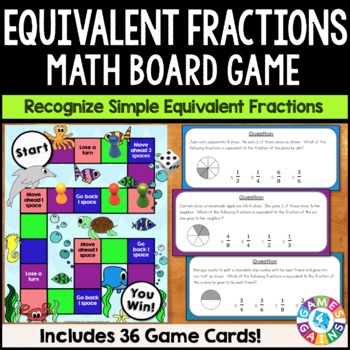# 3rd Grade Equivalent Fractions Game - Word Problem Task Cards Activity

Rated 4.87 out of 5, based on 524 reviews
524 Ratings;
3rd - 4th
Subjects
Resource Type
Standards
Formats Included
• PDF
Pages
28 pages
Report this resource to TPT

#### What educators are saying

This was an awesome activity to add to my lesson. My students really enjoyed and it kept them engaged. Thanks.
Great activity for the students to continue their learning on equivalent fractions. Fun and engaging!
##### Also included in
1. This Fractions Games Bundle makes 3rd grade fraction standards so much fun for students to practice! The games within this bundle cover fraction models, equivalent fractions, comparing fractions, and fractions on a number line. These games are perfect for small groups and centers, or can even be us
Price \$12.60Original Price \$14.75Save \$2.15

### Description

This Equivalent Fractions Board Game contains 36 WORD PROBLEM game cards and a game board to help students practice recognizing and generating equivalent fractions. This game works great as a pair/group activity, or for use in math centers!

This game was created to support these 3rd grade Common Core standards:

CCSS.MATH.CONTENT.3.NF.A.3

Explain equivalence of fractions in special cases, and compare fractions by reasoning about their size.

CCSS.MATH.CONTENT.3.NF.A.3.A

Understand two fractions as equivalent (equal) if they are the same size, or the same point on a number line.

CCSS.MATH.CONTENT.3.NF.A.3.B

Recognize and generate simple equivalent fractions, e.g., 1/2 = 2/4, 4/6 = 2/3. Explain why the fractions are equivalent, e.g., by using a visual fraction model.

CCSS.MATH.CONTENT.3.NF.A.3.C

Express whole numbers as fractions, and recognize fractions that are equivalent to whole numbers. Examples: Express 3 in the form 3 = 3/1; recognize that 6/1 = 6; locate 4/4 and 1 at the same point of a number line diagram.

Included with this Equivalent Fractions Board Game:

• Instructions for play

• Game board

• 36 word problem game cards, addressing the following skills:

→ Determining whether or not two fractions are equivalent based on their size

→ Recognizing and generating simple equivalent fractions with and without visual models

→ Expressing whole numbers as fractions and vice versa

The answers are included on the back of the cards.

Think ahead and save \$\$ by buying this game as part of this FRACTIONS BOARD GAMES BUNDLE, which includes these four exciting games:

Fraction Models Board Game

Fractions on a Number Line Board Game

Equivalent Fractions Board Game

Comparing Fractions Board Game

~~~~~~~~~

You might also like these other games by Games 4 Gains:

3rd Grade Equivalent Fractions Digital Practice

4th Grade Equivalent Fractions Digital Practice

Equivalent Fractions 'Clip and Flip' Cards

Equivalent Fractions Bingo

Fraction Models Board Game

Fractions on a Number Line Board Game

Comparing Fractions Board Game

Comparing Fractions 'Clip and Flip' Cards

~~~~~~~~~

Customer Tips:

We love to hear what you think! Please leave your feedback on this resource to earn credit points to save money on future purchases!

Click on the green ★ above to follow my store to get notifications of new resources, sales, and freebies!

Connect with me on social!

~~~~~~~~~

Created by Brittney Field, © Games 4 Gains, LLC.

This purchase is for single classroom use only. Sharing this resource with multiple teachers, an entire school, or an entire school system is strictly forbidden. Multiple licenses are available at a discount.

Total Pages
28 pages
Included
Teaching Duration
N/A
Report this resource to TPT
Reported resources will be reviewed by our team. Report this resource to let us know if this resource violates TPT’s content guidelines.

### Standards

to see state-specific standards (only available in the US).
Explain equivalence of fractions in special cases, and compare fractions by reasoning about their size.
Understand two fractions as equivalent (equal) if they are the same size, or the same point on a number line.
Recognize and generate simple equivalent fractions, (e.g., 1/2 = 2/4, 4/6 = 2/3). Explain why the fractions are equivalent, e.g., by using a visual fraction model.
Express whole numbers as fractions, and recognize fractions that are equivalent to whole numbers. Examples: Express 3 in the form 3 = 3/1; recognize that 6/1 = 6; locate 4/4 and 1 at the same point of a number line diagram.